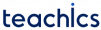# Arithmetic Instructions in 8085

Arithmetic instructions perform various arithmetic operations such as addition, subtraction, increment, and decrement.

Commonly used arithmetic operations are:

• Subtract
• Increment
• Decrement

The complete list of arithmetic instructions in 8085 is described in the following table.

Mnemonic Description Example
Adds the contents of register R to the contents of the accumulator.
Adds the second byte to the contents of the accumulator.
SUB R 1-byte instruction
Subtracts the contents of register R from the contents of the accumulator.
SUB B
SUI 8-bit 2-byte instruction
Subtract the second byte from the contents of the accumulator.
SUI 34H
SUB M Subtracts the content of memory from A; the address of memory is in HL register. SUB M
INR R 1-byte instruction
Increases the contents of register R by 1
Caution: All flags except the CY are affected.
INR D
INR M Increments the content of memory the address of which is in HL register. INR M
DCR R 1-byte instruction
Decreases the contents of register R by 1
Caution: All flags except the CY are affected
DCR B
DCR M Decrements the content of memory the address of which is in HL register. DCR M
INX Rp Increments the content of the register pair. INX H
DCX Rp Decrements the content of the register pair. DCX B

All instructions except INR and DCR,

• Assume that the accumulator is one of the operands.
• Modifies all the flags according to the data conditions of the result.
• Place the result in the accumulator.
• Do not affect the contents of the operand register.

The INR and DCR instructions affect the contents of the specified register and all flags except the CY flag.

Subscribe
Notify of